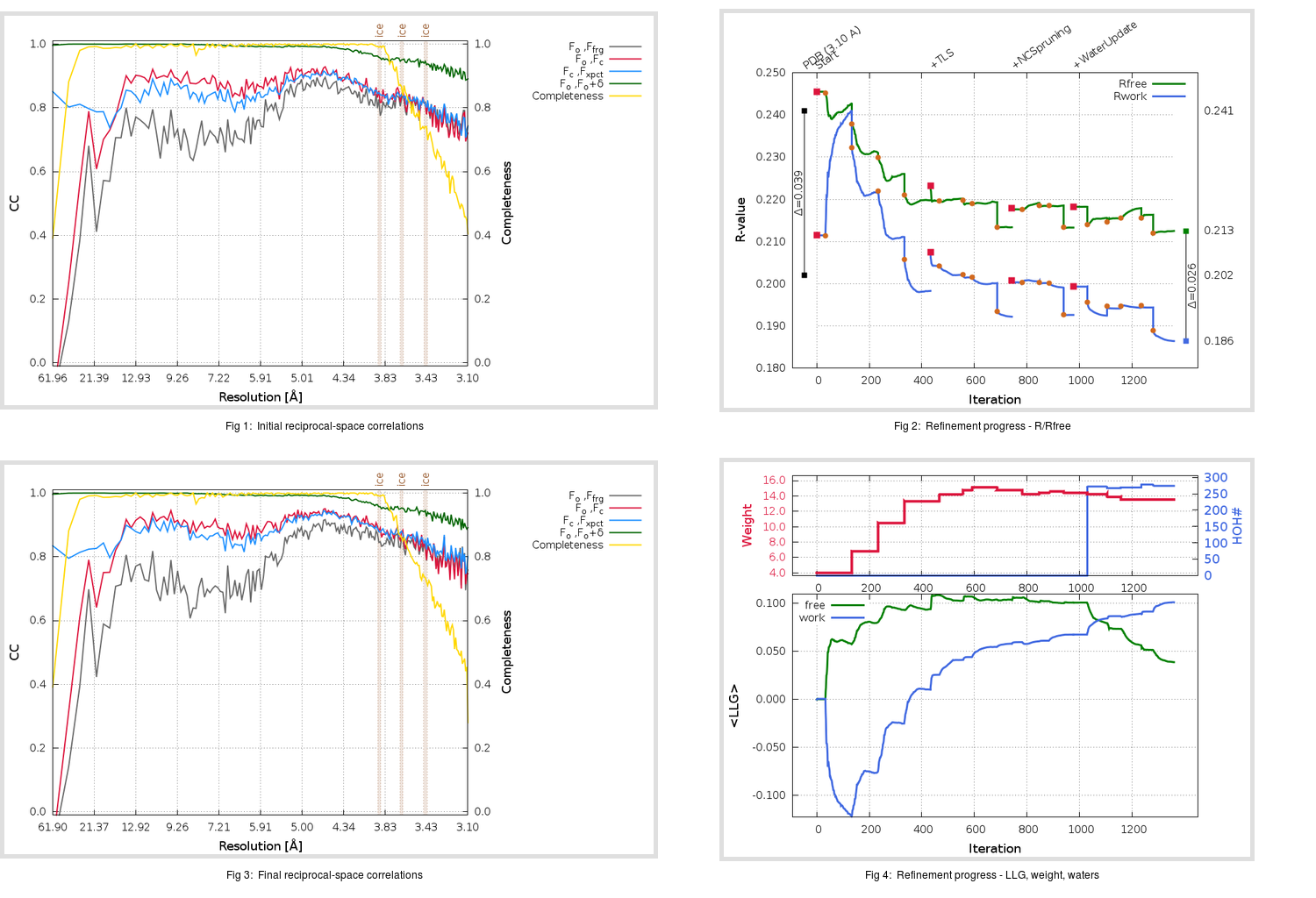Content:

```    Diffraction limits & principal axes of ellipsoid fitted to diffraction cut-off surface:
3.710         0.9904   0.0000  -0.1385       0.850 a* - 0.527 c*
2.933         0.0000   1.0000   0.0000       b*
3.035         0.1385   0.0000   0.9904       0.171 a* + 0.985 c*
```

## Deposited

` `
 Date deposited Date data collection Resolution R, Rfree 20191107 20160611 3.10 0.2000 0.2410

Molprobity (CCP4 7.0 version) summary:

```Ramachandran outliers =   0.03 %
favored =  94.96 %
Rotamer outliers      =   0.39 %
C-beta deviations     =     1
Clashscore            =   6.94
RMS(bonds)            =   0.0039
RMS(angles)           =   0.65
MolProbity score      =   1.73
Resolution            =   3.10
R-work                =   0.2000
R-free                =   0.2410
```

```Number of waters      =     0

<B> (all atoms) =   63.05 ( sd =   20.50 ) for      30730 non-hydrogen atoms
<B>   (protein) =   62.49 ( sd =   20.11 ) for      30056 non-hydrogen atoms
<B>     (water) =    0.00 ( sd =    0.00 ) for          0 non-hydrogen atoms
<B>    (others) =   87.98 ( sd =   22.46 ) for        674 non-hydrogen atoms

B min/max       (all non-hydrogen atoms) =   23.98 /  224.86
B min/max   (protein non-hydrogen atoms) =   23.98 /  224.86
B min/max     (water non-hydrogen atoms) =    0.00 /    0.00
B min/max     (other non-hydrogen atoms) =   35.75 /  146.31
```

## BUSTER (re-)refinement

` `

Molprobity (CCP4 7.0 version) summary:

```Ramachandran outliers =   0.57 %
favored =  93.91 %
Rotamer outliers      =   8.33 %
C-beta deviations     =     6
Clashscore            =   5.21
RMS(bonds)            =   0.0124
RMS(angles)           =   1.66
MolProbity score      =   2.38
Resolution            =   3.10
R-work                =   0.1864
R-free                =   0.2125
```

```Number of waters      =   274

<B> (all atoms) =   70.36 ( sd =   14.69 ) for      31004 non-hydrogen atoms
<B>   (protein) =   70.16 ( sd =   14.36 ) for      30056 non-hydrogen atoms
<B>     (water) =   50.13 ( sd =   11.75 ) for        274 non-hydrogen atoms
<B>    (others) =   87.41 ( sd =   14.64 ) for        674 non-hydrogen atoms

B min/max       (all non-hydrogen atoms) =   17.42 /  138.62
B min/max   (protein non-hydrogen atoms) =   45.09 /  138.62
B min/max     (water non-hydrogen atoms) =   17.42 /  104.53
B min/max     (other non-hydrogen atoms) =   50.26 /  117.07
```

Refinement progression:Results:

` `
 File Remark 6L8Q_aB_refine.01_04_refine.pdb.gz exact refinement commands are in header 6L8Q_aB_refine.01_04_refine.mtz.gz including original deposited data and several re-refinement map coefficients 6L8Q_aB_refine.01_04_BUSTER_model.cif.gz including any non-standard compound restraints 6L8Q_aB_refine.01_04_BUSTER_refln.cif.gz